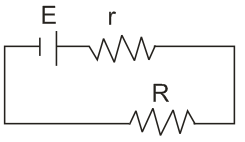Q

# Stuck here, help me understand: A cell of internal resistance r drives current through an external resistance R. The power delivered by the cell to the external resistance will be maximum when:

A cell of internal resistance r drives current through an external resistance R. The power delivered by the cell to the external resistance will be maximum when:

• Option 1)

R=0.001 r

• Option 2)

R=1000 r

• Option 3)

R=2r

• Option 4)

R=r

ViewsP across

For maximum=

R=r

Option 1)

R=0.001 r

Option 2)

R=1000 r

Option 3)

R=2r

Option 4)

R=r

Exams
Articles
Questions